• Maxout-PyTorch 这是纸上的Maxout层的实现：PyTorch中的 。 在前向传递中，计算CNN特征图的maxout。 对于后向传递，错误wrt输入的导数仅通过在正向传递中激活的特征图数组的单元传播（这是Maxout的导数）。 为确保...
• 针对深度卷积神经网络中 maxout 单元非最大特征无法传递、特征图像子空间池化表达能力不足的局限性，提出混合maxout (mixout,mixed maxout)单元。首先，计算相同输入在不同卷积变换下所形成的特征图像子空间的指数...深度学习 卷积神经网络 激活函数
• Convolutional Maxout Neural Networks for Speech Separation研究论文
• ## Maxout 论文笔记

千次阅读 2018-05-09 21:49:21
MaxoutMaxout networks Maxout模型实际上是一种新型的激活函数。 maxout的思想其实和dropout有点像，都是多个不同训练模型的集成技术。 1.Dropout dropout由Hinton提出的一个网络正则技术。dropout通过对共享...
Maxout：Maxout networks
Maxout模型实际上是一种新型的激活函数。
maxout的思想其实和dropout有点像，都是多个不同训练模型的集成技术。
1.Dropout dropout由Hinton提出的一个网络正则技术。dropout通过对共享参数的fc层的一些神经元固定概率的随机置零，来集成多个模型，并且对多个模型的预测进行了平均。dropout是一个通用技术，可以用于任何网络，但是dropout的模型平均能力并没有被证明。使用了dropout的训练过程和一般的SGD方法完全不同。dropout在更新时使用更大的步长最有效，因为这样可以在不同的训练子集上对不同的模型有明显的影响来使得目标函数有持续的波动性，理想情况下整个训练过程就类似于使用bagging来训练集成的模型（带有参数共享的约束）。而一般的SGD更新时会使用更小的步长，来使得目标函数平滑的下降。对于深度网络模型，dropout只能作为模型平均的一种近似，显式的设计模型来最小化这种近似误差也可以提高dropout的性能[参考文献]。
dropout训练的集成模型中，所有模型都只包括部分输入和部分隐层参数。对每一个训练样本，我们都会训练一个包括不同隐层参数的子模型。dropout与bagging的相同点是不同的模型使用不同数据子集，不同点是dropout的每个模型都只训练一次且所有模型共享参数。这一段有争议
对于预测时如何平均所有子模型的问题，bagging一般使用的是算数平均，而对dropout产生的指数多个子模型则并非显而易见。但是如果模型只有一层，则最终的预测分布就是简单的指数多个子模型的算数平均。这个结果只能用在单层模型中，如果是深层模型，则dropout产生的指数个模型的集成更复杂，将由单层的算数平均转为几何平均。这一段有争议
2.Maxout 使用maxout的默认先验：样本集是凸集可分的 Maxout其实是一种新的激活函数。在前馈式神经网络中，Maxout的输出取该层的多个fc的同一位置的神经元的最大值；卷积中，Maxout的输出取多个feature map同一位置的最大值。这句话需要好好理解
我们知道，多层感知器（MLP）是一种通用的函数拟合器，也就是说它可以拟合任意复杂的函数，只不过有些函数使用MLP来训练比较麻烦而已。
而Maxout模型恰好是一种基于MLP的激活函数，因为每个Maxout模型内部可以包含任意的仿射变换，这就导致了Maxout模型也是一个通用的函数拟合器。
例如：下图是一个包含4个神经元的Maxout网络。从输入层到Maxout的输出，是经过了三个仿射变换，我们可以认为这三个仿射变换就是三个特征提取器，此变换中没有任何非线性激活函数，完全是一个线性变换，最终由这三个变换取最大值得到Maxout的4个输出神经元。
根据上图，我们可以看到，假如输入变量的维度是2，那么Maxout中的权重变量就是4维，因为多余的两个维度分别是Maxout自身神经元的个数以及特征提取器的个数。
我们再回到前面所说的Maxout的通用拟合的功能，下图是原始论文中出现的一张图，其目的是告诉我们无论是ReLU函数，还是V型函数还是二次型函数，我们都可以通过构造多个仿射变换来对其进行逼近，并且在每个区间内取其最大值即可。这不就是Maxout的功能吗？虽然这里只给出了二维函数的逼近示意图，但是实际上Maxout可以拟合任意的凸函数。maxout激活函数的数学描述：

h

i

(

x

)

=

max

⁡

j

∈

[

1

,

k

]

z

i

j

h_{i}(x)=\max\limits_{j\in{[1,k]}}{z_{ij}}

在MLP中：

z

i

j

=

x

T

W

.

.

.

i

j

+

b

i

j

z_{ij}=x^{T}W_{...ij}+b_{ij}

其中

W

∈

R

d

×

m

×

k

W\in{\mathbb{R}^{d\times{m}\times{k}}}

，

b

∈

R

m

×

k

b\in{\mathbb{R}^{m\times{k}}}

在卷积网络中：

z

i

j

z_{ij}

为各个feature map
maxout网络可以拟合任意连续函数，只要maxout网络中有足够多的

z

i

j

z_{ij}

。
maxout网络不仅可以学习到k个

z

i

j

z_{ij}

之间的关系，还可以学习到maxout每个输出单元的激活函数。
maxout放弃了传统激活函数的设计，它产生的表示不再是稀疏的，但是它的梯度是稀疏的，且dropout可以将它稀疏化。
maxout没有上下界，所以让它在某一端饱和是零概率事件。
如果训练时使用dropout，则dropout操作在矩阵相乘之前，而并不对max操作的输入执行dropout。
3.Dropout和Maxout结合 Dropout和Maxout结合起来效果会更好。 结合时，应该在矩阵相乘之前使用dropout，而并不对max操作的输入执行dropout
4.Maxout的各种实现： 1.Tensorflow内部的Maxout激活函数：
# 代码来自于tensorflow.contrib.layers.maxout
import tensorflow as tf
def maxout(inputs, num_units, axis=-1, scope='Maxout'):
'''
inputs: Tensor input
num_units: The num of unit keeped after amxout
axis: The dimension max op performed
scope: Optional scope for variable_scope
注意：这是略加修改的版本。替换了一些不常用的API函数
'''
with tf.variable_scope(scope):
shape = inputs.get_shape().as_list()
num_channels = shape[axis]
if num_channels % num_units:
raise ValueError('number of features({}) is not '
'a multiple of num_units({})'.format(
num_channels, num_units))
shape[axis] = -1
shape += [num_channels // num_units]

# Dealing with batches with arbitrary sizes
for i in range(len(shape)): # 这里用来处理shape中包含None的情况
if shape[i] is None:
shape[i] = tf.shape(inputs)[i]
outputs = tf.reduce_max( tf.reshape(inputs, shape), -1, keepdims=False)
return outputs

上面是绝对正确的TF实现，其对应文章中的

h

i

(

x

)

=

max

⁡

j

∈

[

1

,

k

]

h_{i}(x)=\max\limits_{j\in{[1,k]}}

，inputs对应

z

i

j

z_{ij}

，并不包含

z

i

j

z_{ij}

的计算。
2.Keras里MLP版Maxout层的实现（该实现现在已经被去除掉了）
# maxout 网络层类的定义
# 这里的MaxoutDense只是Maxout在MLP中的实施
class MaxoutDense(Layer):
# 网络输入数据矩阵大小为(nb_samples, input_dim)
# 网络输出数据矩阵大小为(nb_samples, output_dim)
input_ndim = 2
#nb_feature就是我们前面说的k的个数了，这个是maxout层特有的参数
def __init__(self, output_dim, nb_feature=4,
init='glorot_uniform', weights=None,
W_regularizer=None, b_regularizer=None, activity_regularizer=None,
W_constraint=None, b_constraint=None, input_dim=None, **kwargs):
self.output_dim = output_dim
self.nb_feature = nb_feature
self.init = initializations.get(init)

self.W_regularizer = regularizers.get(W_regularizer)
self.b_regularizer = regularizers.get(b_regularizer)
self.activity_regularizer = regularizers.get(activity_regularizer)

self.W_constraint = constraints.get(W_constraint)
self.b_constraint = constraints.get(b_constraint)
self.constraints = [self.W_constraint, self.b_constraint]

self.initial_weights = weights
self.input_dim = input_dim
if self.input_dim:
kwargs['input_shape'] = (self.input_dim,)
self.input = K.placeholder(ndim=2)
super(MaxoutDense, self).__init__(**kwargs)
#参数初始化部分
def build(self):
input_dim = self.input_shape

self.W = self.init((self.nb_feature, input_dim, self.output_dim))#nb_feature是我们上面说的k。
self.b = K.zeros((self.nb_feature, self.output_dim))

self.params = [self.W, self.b]
self.regularizers = []

if self.W_regularizer:
self.W_regularizer.set_param(self.W)
self.regularizers.append(self.W_regularizer)

if self.b_regularizer:
self.b_regularizer.set_param(self.b)
self.regularizers.append(self.b_regularizer)

if self.activity_regularizer:
self.activity_regularizer.set_layer(self)
self.regularizers.append(self.activity_regularizer)

if self.initial_weights is not None:
self.set_weights(self.initial_weights)
del self.initial_weights

def get_output(self, train=False):
X = self.get_input(train)#需要切记这个x的大小是(nsamples,input_num)
# -- don't need activation since it's just linear.
output = K.max(K.dot(X, self.W) + self.b, axis=1)#maxout激活函数
return output

上面代码其实最主要的一句是：
output = K.max(K.dot(X, self.W) + self.b, axis=1)

注意Keras和TF里Maxout实现的不同，TF实现了一个通用的maxout激活函数，keras里将论文中的

z

i

j

z_{ij}

的计算也包含到了代码实现中。
最后附上一些网上的实现，但是都没有TF里实现的好，TF里的maxout更加通用。
# 实现1
def maxout(x, k, m):
# 这个实现只适用于MLP
d = x.get_shape().as_list()[-1]
W = tf.Variable(tf.random_normal(shape=[d, m, k]))
b = tf.Variable(tf.random_normal(shape = [m, k]))
z = tf.tensordot(x, W, axes=1) + b
z = tf.reduce_max(z, axis=2)
return z

# 实现2
def maxout_cnn_layer(inputs, filter, bind_num,strides, padding, name=None):
# 这个实现只适用于卷积层
return maxout(conv, bind_num)

def maxout_weights(filter_shape,bind_num):
filter_shape[- 1]=filter_shape[- 1]*bind_num
return tf.Variable(tf.random_normal(filter_shape, stddev= 0.01))

def maxout(inputs, bind_num):
shape = inputs.get_shape().as_list()
in_chan = shape[- 1]
shape[- 1] = in_chan//bind_num
shape += [bind_num]
shape[ 0]=- 1
return tf.reduce_max(tf.reshape(inputs,shape),- 1,keep_dims= False)

maxout网络的tensorflow实现（数据集：MNIST）
import tensorflow as tf
maxout = tf.contrib.layers.maxout
'''
未来如果maxout激活函数被TF去除，读者将上面的maxout激活函数在TF的实现搬下来即可。
network configuration comes from goodfellow's github
'''

def inference(inputs,
num_classes=10,
is_training=True,
dropout_keep_prob=0.5,
scope='inference'):

x = inputs
with tf.variable_scope('maxout1'):
x = maxout(x, 4) # 2
with tf.variable_scope('maxout2'):
x = maxout(x, 4) # 2
with tf.variable_scope('maxout3'):
x = maxout(x, 8) # 4
with tf.variable_scope('fc'):
_ksize = x.get_shape().as_list()
logits = tf.layers.Conv2D(num_classes, [_ksize, _ksize], activation=tf.nn.softmax)(x)
logits = tf.layers.flatten(logits)
return logits

if __name__ == '__main__':
batch_size = 64
x = tf.placeholder(tf.float32, [batch_size, 784])
images = tf.reshape(x,[-1,28,28,1])
labels = tf.placeholder(tf.float32, [batch_size, 10])

logits = inference(inputs=images, num_classes=10)
print('inference is ok!')

上面的代码只给出了inference部分。网络的参数来自于于作者Goodfellow的github，作者使用的是pylearn2框架。这里对作者的参数做了一点点修改，修改的地方注释了原始参数。
注意：使用本博客的代码，请添加引用
参考文献： Maxout网络学习
展开全文• ## Maxout

2015-11-25 00:51:51
Reading Paper-Network In Network(1)-MaxoutThe maxout is designed to solve the problem that the representations that achieve good abstraction are generally highly nonlinear functions of the input data.
Reading Paper-Network In Network(1)-Maxout
The maxout is designed to solve the problem that the representations that achieve good abstraction are generally highly nonlinear functions of the input data. Generally, the convolutional layers generate feature maps by liner convolutional filters followed by nonlinear activation functions. the feature map can be calculated as follows:here it is a generalized linear model(GLM). However, some papers points that replacing the GLM with nonlinear function approximator can enhance the performance.  1 Maxout Network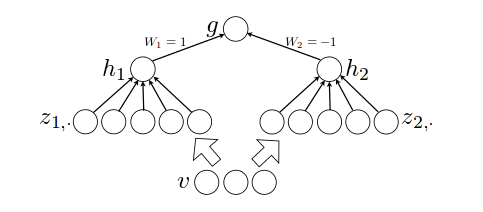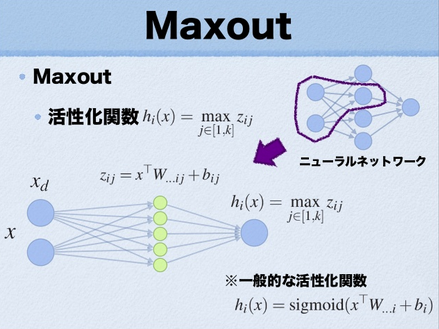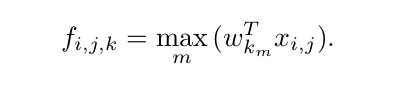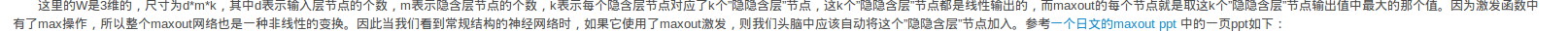For the maxout network, it adds another network between adjacent layers witch performs nonliner function to input data.
展开全文• ## Maxout详解

万次阅读 多人点赞 2018-09-21 11:53:35
本篇博文主要讲解2013年，ICML上的一篇文献：《Maxout Networks》，这个算法我目前也很少用到，个人感觉最主要的原因应该是这个算法参数个数会成k倍增加(k是maxout的一个参数)，不过没关系，对于我们来说知识积累...
一、相关理论
本篇博文主要讲解2013年，ICML上的一篇文献：《Maxout  Networks》，这个算法我目前也很少用到，个人感觉最主要的原因应该是这个算法参数个数会成k倍增加(k是maxout的一个参数)，不过没关系，对于我们来说知识积累才是最重要的，指不定某一天我们就需要用到这个算法，技多不压身。个人感觉Maxout网络和Dropout有很多相似的地方。
本篇博文将从什么是maxout网络讲起，先解答maxout的源码层实现，因为很多人最感兴趣的还是算法要怎么实现，当然我也是这样的。我看文献，一般最在意的还是源码的实现，有的文献理论公式推导了十几页，结果5行代码搞定，我看到想哭，这也许就是我讨厌做学术研究的原因吧。知道了源码怎么实现后，我们简单啰嗦一下maxout相关的理论意义。
二、Maxout算法流程
1、算法概述
开始前我们先讲解什么叫maxout networks，等我们明白了什么叫maxout 网络后，再对maxout的相理论意义做出解释。Maxout是深度学习网络中的一层网络，就像池化层、卷积层一样等，我们可以把maxout 看成是网络的激活函数层，这个后面再讲解，本部分我们要先知道什么是maxout。我们假设网络某一层的输入特征向量为：X=（x1,x2,……xd），也就是我们输入是d个神经元。Maxout隐藏层每个神经元的计算公式如下：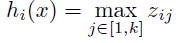上面的公式就是maxout隐藏层神经元i的计算公式。其中，k就是maxout层所需要的参数了，由我们人为设定大小。就像dropout一样，也有自己的参数p(每个神经元dropout概率)，maxout的参数是k。公式中Z的计算公式为：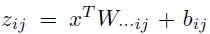权重w是一个大小为(d,m,k)三维矩阵，b是一个大小为(m,k)的二维矩阵，这两个就是我们需要学习的参数。如果我们设定参数k=1，那么这个时候，网络就类似于以前我们所学普通的MLP网络。
我们可以这么理解，本来传统的MLP算法在第i层到第i+1层，参数只有一组，然而现在我们不怎么干了，我们在这一层同时训练n组参数，然后选择激活值最大的作为下一层神经元的激活值。下面还是用一个例子进行讲解，比较容易搞懂。
为了简单起见，假设我们网络第i层有2个神经元x1、x2，第i+1层的神经元个数为1个，如下图所示：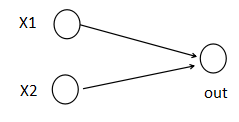(1)以前MLP的方法。我们要计算第i+1层，那个神经元的激活值的时候，传统的MLP计算公式就是：
z=W*X+b
out=f(z)
其中f就是我们所谓的激活函数，比如Sigmod、Relu、Tanh等。
(2)Maxout 的方法。如果我们设置maxout的参数k=5，maxout层就如下所示：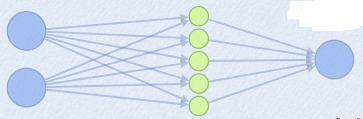相当于在每个输出神经元前面又多了一层。这一层有5个神经元，此时maxout网络的输出计算公式为：
z1=w1*x+b1
z2=w2*x+b2
z3=w3*x+b3
z4=w4*x+b4
z5=w5*x+b5
out=max(z1,z2,z3,z4,z5)
所以这就是为什么采用maxout的时候，参数个数成k倍增加的原因。本来我们只需要一组参数就够了，采用maxout后，就需要有k组参数。
三、源码实现
ok，为了学习maxout源码的实现过程，我这边引用keras的源码maxout的实现，进行讲解。keras的网站为：http://keras.io/   。项目源码网站为：https://github.com/fchollet/keras。下面是keras关于maxout网络层的实现函数：
#maxout 网络层类的定义
class MaxoutDense(Layer):
# 网络输入数据矩阵大小为(nb_samples, input_dim)
# 网络输出数据矩阵大小为(nb_samples, output_dim)
input_ndim = 2
#nb_feature就是我们前面说的k的个数了，这个是maxout层特有的参数
def __init__(self, output_dim, nb_feature=4,
init='glorot_uniform', weights=None,
W_regularizer=None, b_regularizer=None, activity_regularizer=None,
W_constraint=None, b_constraint=None, input_dim=None, **kwargs):
self.output_dim = output_dim
self.nb_feature = nb_feature
self.init = initializations.get(init)

self.W_regularizer = regularizers.get(W_regularizer)
self.b_regularizer = regularizers.get(b_regularizer)
self.activity_regularizer = regularizers.get(activity_regularizer)

self.W_constraint = constraints.get(W_constraint)
self.b_constraint = constraints.get(b_constraint)
self.constraints = [self.W_constraint, self.b_constraint]

self.initial_weights = weights
self.input_dim = input_dim
if self.input_dim:
kwargs['input_shape'] = (self.input_dim,)
self.input = K.placeholder(ndim=2)
super(MaxoutDense, self).__init__(**kwargs)
#参数初始化部分
def build(self):
input_dim = self.input_shape

self.W = self.init((self.nb_feature, input_dim, self.output_dim))#nb_feature是我们上面说的k。
self.b = K.zeros((self.nb_feature, self.output_dim))

self.params = [self.W, self.b]
self.regularizers = []

if self.W_regularizer:
self.W_regularizer.set_param(self.W)
self.regularizers.append(self.W_regularizer)

if self.b_regularizer:
self.b_regularizer.set_param(self.b)
self.regularizers.append(self.b_regularizer)

if self.activity_regularizer:
self.activity_regularizer.set_layer(self)
self.regularizers.append(self.activity_regularizer)

if self.initial_weights is not None:
self.set_weights(self.initial_weights)
del self.initial_weights

def get_output(self, train=False):
X = self.get_input(train)#需要切记这个x的大小是(nsamples,input_num)
# -- don't need activation since it's just linear.
output = K.max(K.dot(X, self.W) + self.b, axis=1)#maxout激活函数
return output

看上面的代码的话，其实只需要看get_output()函数，就知道maxout的实现了。所以说有的时候，一篇文献的代码，其实就只有几行代码，maxout就仅仅只有一行代码而已：
output = K.max(K.dot(X, self.W) + self.b, axis=1)#maxout激活函数
下面在简单啰嗦一下相关的理论，毕竟文献的作者写了那么多页，我们总得看一看才行。Maxout可以看成是一个激活函数 ，然而它与原来我们以前所学的激活函数又有所不同。传统的激活函数：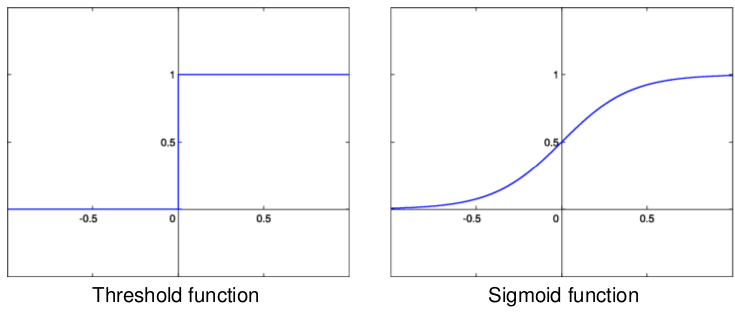比如阈值函数、S函数等。maxout激活函数，它具有如下性质：
1、maxout激活函数并不是一个固定的函数，不像Sigmod、Relu、Tanh等函数，是一个固定的函数方程
2、它是一个可学习的激活函数，因为我们W参数是学习变化的。
3、它是一个分段线性函数：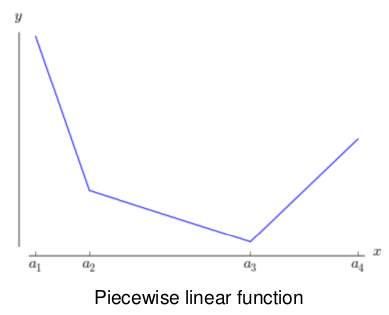然而任何一个凸函数，都可以由线性分段函数进行逼近近似。其实我们可以把以前所学到的激活函数：relu、abs激活函数，看成是分成两段的线性函数，如下示意图所示：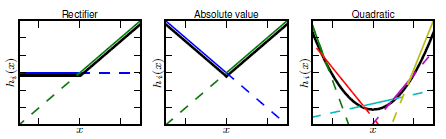maxout是一个函数逼近器，对于一个标准的MLP网络来说，如果隐藏层的神经元足够多，那么理论上我们是可以逼近任意的函数的。类似的，对于maxout 网络也是一个函数逼近器。
定理1：对于任意的一个连续分段线性函数g(v)，我们可以找到两个凸的分段线性函数h1(v)、h2(v)，使得这两个凸函数的差值为g(v)：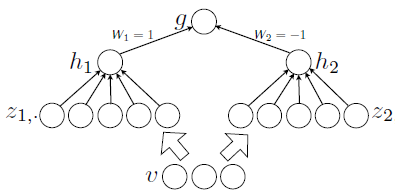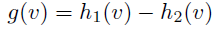参考文献：
1、《Maxout  Networks》
原文地址：http://blog.csdn.net/hjimce/article/details/50414467
作者：hjimce
展开全文• ## 激活函数maxout

万次阅读 2020-03-18 19:56:22
激活函数maxout系列文章：   maxout函数相对于其他的激活函数有很大的区别，可以看做是在神经网络中激活函数的地方加入一个激活函数层。maxout可以看做是一个可学习的分段线性函数，因为可学习所以是需要参数的，...


激活函数maxout
系列文章：

maxout函数相对于其他的激活函数有很大的区别，可以看做是在神经网络中激活函数的地方加入一个激活函数层。
maxout可以看做是一个可学习的分段线性函数，因为可学习所以是需要参数的，而且参数是可以通过反向传播来学习的。因为参数量的增大，势必导致计算量的增大。
传统的神经网络从第i层输入到第i+1层，只需要训练一组权重参数，这些参数决定了上一层输入到到达这一层之后进行的变换。但是maxout做的事情就是第i层到第i+1层之前我们多训练一些参数，对第i层的输出，我们将每个输出连接k个隐藏层，通过k个隐藏层的参数进行计算之后，然后把这k个隐藏层求最大值，作为最终的输出。

这里我们给出maxout的计算公式。，使用maxout需要人为的设定一个参数k，这个参数k就是每个神经元输出之后接的虚拟隐藏层的个数。而权重参数W的维度是

(

d

,

m

,

k

)

(d,m,k)

的，d代表的是输入节点的个数，m代表的则是输出层节点的个数。这也是之前神经网络第i+1层的参数设置。但是现在多出来一个维度k，就是为了产生中间的k个输出，然后将这k个输出再取一个最大值。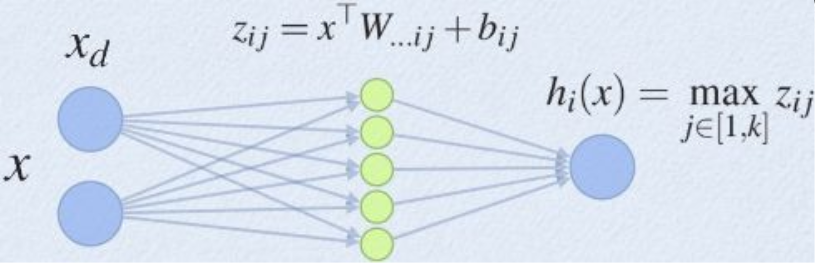如上图，原本的神经网络只有输入的两个神经元x，输出的只有一个神经元

h

i

(

x

)

h_i(x)

，普通的神经元则是直接把x和

h

i

(

x

)

h_i(x)

连接，现在相当于在两层之间又增加了

k

=

5

k=5

个参数，然后输入先经过这个maxout的隐藏层得到输出，然后对输出取最大值，再输入到下一层当中。其中虚拟隐藏层的

W

W

和

b

b

就是所需要学习的参数。可以看到，参数量的增大是针对每一个输出神经元增加k个的。   普通的神经元配合激活函数得到一组输出，经过了maxout将会得到k组输出，然后在k组之中取最大值。可见maxout非线性的拟合能力是更强的，因为参数更多，而且可学习。我们能想到

r

e

l

u

(

z

)

=

m

a

x

(

0

,

z

)

relu(z)=max(0,z)

，而maxout也是取最大，如果我们能让一个虚拟神经元学习

m

(

x

)

=

x

m(x)=x

，一个虚拟神经元学习

m

(

x

)

=

0

m(x)=0

，然后两个神经元求最大就得到了relu激活函数。可见这是k=2的情况，如果我们有更多的k，就可以学习更加负责的激活函数，这就是maxout拟合能力更强的原因。事实上maxout可以拟合任意的凸函数。   相比于ReLU，ReLU有的优点maxout有，是分段线性的，不容易梯度消失。同时ReLU没有的优点，maxout可能也有，比如神经元不会死亡。但是这些优点是通过计算量换来的，maxout的计算量非常大，我们已经解释过。   这个激活函数的实现没什么难的，就是在指定的维度上取最大值即可，但是为了得到能够得到这个要取最大值的维度的变量，往往需要在上一层的神经元上做手脚，需要增加参数量。原本是m维的输出通道数，现在需要变成k×m的数出通道。给出tensorflow代码示例。
x=tf.random_normal([1,3])
m=4  # out_channel
k=3  # maxout_chanenl
d=x.get_shape().as_list()[-1]
W=tf.Variable(tf.random_normal(shape=[d,m,k]))
b=tf.Variable(tf.random_normal(shape=[m,k]))
# 从W和b的维度看出参数量是增大了
dot_z=tf.tensordot(x,W,axes=1)+b
z=tf.reduce_max(dot_z,axis=2)  # 这里再把maxout的通道给取最大降维下去

with tf.Session() as sess:
sess.run(tf.global_variables_initializer())
print(sess.run([x,dot_z,z]))

事实上这里还有一种方式就是为maxout特殊定义一个方法或者类，然后就可以像其他普通的激活函数一样使用。以下给出tensorflow代码示例。
def maxout(inputs, num_units, axis=None):
'''
inputs: 上一层的输出的结果，这里需要上一层的输出结果的维度是k*m
num_units:激活后的最终的输出结果数m
'''
shape = inputs.get_shape().as_list()
if axis is None:
# Assume that channel is the last dimension
axis = -1
num_channels = shape[axis]
if num_channels % num_units:
raise ValueError('number of features({}) is not a multiple of num_units({})'
.format(num_channels, num_units))
shape[axis] = num_units
shape += [num_channels // num_units]
for i in range(len(shape)):
if shape[i] is None:
shape[i] = -1
outputs = tf.reduce_max(tf.reshape(inputs, shape), -1, keepdims=False)
return outputs

# X.shape = (..., d)
X = tf.layers.conv2d(inputs=X, filters=k * m, kernel_size, strides, padding)
# X.shape = (..., m*k)
X = maxout(inputs=X, num_units=m)
# X.shape = (..., m)

系列文章：
神经网络中的激活函数总述 sigmoid激活函数 tanh激活函数 ReLU系列激活函数 maxout激活函数 Swish激活函数 激活函数发展的新里程——EvoNorms
展开全文神经网络 深度学习 激活函数
• 本篇blog内容基于Maxout Networks(Goodfellow, Yoshua Bengio, 2013)。在GAN很多应用中都用到了这个技巧。 全篇大部分转载自：Maxout Networks， 深度学习（二十三）Maxout网络学习 作者：hjimce  一、Dropout ...GAN
• 我们定义了一个简单的新模型maxout(之所以这样命名，是因为它的输出是一组输入的最大值，而且它是dropout的一个自然伙伴)，旨在促进dropout优化和提高dropout的快速近似模型平均技术的准确性。我们通过经验验证模型...计算机视觉 深度学习 机器学习
• Maxout神经元有望成为sigmod神经元的替代品。 通过选择局部区域内的最大值来获得maxout神经元的激活，从而在训练过程中产生恒定的梯度。 在本文中，我们将maxout神经元与两个流行的DNN结构进行声学建模，即卷积神经...
• MAXOUT神经网络及实例应用 一、maxout 神经网络的介绍 Maxout 神经网络 可以理解为单个神经元的拓展，主要是拓展单个神经元里面的激活函数。将激活函数变成一个网络选择器，原理就是将多个神经元并排的放在一起，从...
• ## pytorch maxout实现

千次阅读 2020-05-12 09:01:26
看了半天，在网上没有看到pytorch关于maxout的实现。（虽然看到其他的模型也是可以用的，但是为了更好的复现论文，这里还是打算实现下）。 （不一定保证完全正确，估计很快pytorch就会自己更新，对应的maxout激活...深度学习 人工智能 python 神经网络
• Maxout网络学习原文地址：http://blog.csdn.net/hjimce/article/details/50414467作者：hjimce一、相关理论 本篇博文主要讲解2013年，ICML上的一篇文献：《Maxout Networks》，这个算法我目前也很少用到，个人感觉...
• matlab精度检验代码Maxout Networks 该目录包含maxout单元的实现，并提供有关CIFAR-10的简单分类示例。 它基于张家仁的代码，可以找到。 安装 该模块可以与MatConvNet vl_contrib软件包管理器一起安装： vl_contrib...
• 1.Maxout可以与Dropout同时使用提升网络性能 Dropout是一种网络规则化技巧，其实它就是相当于在训练很多个不同的网络结构，尽管如此，推理阶段所有不同结构的参数依然是共享的，因为实际上只有一个网络存在。 在机器...深度学习
• ## 深度学习maxout单元

千次阅读 2018-10-03 16:19:57深度学习
• Maxout 是 Goodfellow 在 2013 年提出的一个新的激活函数，相比于其它的激活函数，Maxout 本身是需要参数的，参数可以通过网络的反向传播得到学习，相应地，它比其它激活函数有着更好的性能，理论上可以拟合任意凸...
• 本文先介绍两个13，14年的工作：Maxout Networks，Network In Network。网上有不少资料，但是很多作者我相信自己都没有完全理解，在本文中我会尽可能描述清楚。本文重点在于Network In Network。本文针对论文和网络...神经网络 深度学习 NIN
• ## 深度学习（二十三）Maxout网络学习

万次阅读 多人点赞 2015-12-27 22:45:16
Maxout网络学习 Maxout是深度学习网络中的一层网络，就像什么激活函数层、池化层、卷积层一样。我们假设网络某一层的输入特征向量为：X=（x1,x2,……xd），也就是我们输入是d个神经元。Maxout隐藏层每个神经元的...
• MaxOut 网络4.1 与ReLU的关系4.2 可能训练处激活函数的样子4.3 MaxOut 的训练 1 sigmoid激活函数的不足之处 在网络结构非常深的情况下，由于梯度消失问题的存在，可能会导致靠近输入附近的隐藏层的权重变化较慢，...
• maxout = tf.reduce_max(z ,axis =1, keep_dims = True) # 设置学习参数 w2 = tf.Variable(tf.truncated_normal([1,10], stddev=0.1)) b2 = tf.Variable(tf.zeros()) # 构建模型 # 损失函数 cost = tf.nn.softmax...
• ## 理解maxout激活函数

千次阅读 2019-05-24 19:40:33
深度学习（二十三）Maxout网络学习 深度学习笔记–激活函数：sigmoid，maxout A Light CNN for Deep Face Representation with Noisy Labels 以上两篇博客都是针对全连接网络来列举的例子，注意在卷积神经网络中是...激活函数
• gta-maxout是动态链接库，最初是为修改游戏而编写的：侠盗猎车手：自由状态。 目前，我正在将源代码转换为可用于侠盗猎车手：罪恶都市的所有Total Conversion修改，也可作为C ++开发人员在游戏引擎内部使用的API。 ...C++
• maxout是Goodfellow设计的一种类似于dropout的网络结构，该网络结构可以更容易的被优化，并且效果也相当可观。 maxout模型通常是一个简单的前馈神经网络，可以用在多层感知机里，也也可以用在卷积神经网络里。 ...神经网络 深度学习 机器学习
• 2.3 Decoding with Attention and Maxout Pointer 使用RNN ${d_t} = RN{N^D}({d_{t - 1}},{y_{t - 1}})$ $p({y_t}|\{ {y_{ ) = soft\max ({W^V}{d_t})$ 注意此处的$$y_t$$不作为最后的输出，还要经过一系列...python 算法 java 机器学习 人工智能
• ## maxout实现-TensorFlow

千次阅读 2018-05-21 15:37:30
1.原理maxout作为一种独特的激活函数，来源于paper Goodfellow, I. J., Warde-Farley, D., Mirza, M., Courville, A., &amp; Bengio, Y. (2013). Maxout networks. arXiv preprint arXiv:1302.4389。这种激活...
• 一、相关理论    本篇博文主要讲解2013年，ICML上的一篇文献：《Maxout  Networks》，这个算法我目前也很少用到，个人感觉最主要的原因应该是这...深度学习 神经网络 人工智能 python
• Maxout可以看做是在深度学习网络中加入一层激活函数层,包含一个参数k.这一层相比ReLU,sigmoid等,其特殊之处在于增加了k个神经元,然后输出激活值最大的值. 我们常见的隐含层节点输出： 而在Maxout网络中，其...激活函数...